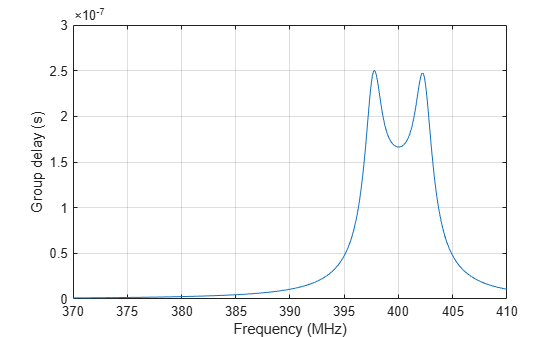# Design IF Butterworth Bandpass Filter

This example shows how to design an Intermediate Frequency (IF) Butterworth bandpass filter with a center frequency of 400 MHz, bandwidth of 5 MHz, and Insertion Loss (IL) of 1dB .

### Account for Mismatch/Insertion Loss (IL)

Practical circuits suffer a certain degree of mismatch. Mismatch happens when an unmatched circuit is connected to an RF source leading to reflections that result in a loss of power delivered to the circuit. You can use IL to define this mismatch. Calculate the output impedance mismatch to account for the given IL. The IL and normalized output impedance (Zout) are related as follows ,:

IL (dB) = -10*log10(1-| ${\gamma }_{in}$ |^2) = -10*log10(4*Zout/(1+Zout)^2)

The roots of the resulting polynomial return the value of normalized Zout. The unnormalized values for Zout are 132.986 Ohms and 18.799 Ohms. Choose the higher value for the filter design to account for the IL.

`Zout = 132.986;`

### Design Filter

Use `rffilter` to design the filter for the desired specifications.

```Fcenter = 400e6; Bwpass = 5e6; if_filter = rffilter('ResponseType','Bandpass', ... 'FilterType','Butterworth','FilterOrder',4, ... 'PassbandAttenuation',10*log10(2), ... 'Implementation','Transfer function', ... 'PassbandFrequency',[Fcenter-Bwpass/2 Fcenter+Bwpass/2],'Zout',Zout);```

### Plot S-parameters and Group Delay of Filter

Calculate sparameters.

```freq = linspace(370e6,410e6,2001); Sf = sparameters(if_filter, freq); figure; rfplot(Sf);```A data cursor showing 1dB IL at Fcenter = 400 MHz

Calculate groupdelay:

```gd = groupdelay(if_filter, freq); figure; plot(freq/1e6, gd); xlabel('Frequency, MHz'); ylabel('Group delay'); grid on;```### Represent Filter as Touchstone File

Use `rfwrite` to write the sparameters of the designed filter for the desired frequency range. You can read this Touchstone file into a `nport` object, which in turn can be inserted into an `rfbudget` object.

```filename = 'filterIF.s2p'; if exist(filename,'file') delete(filename) end rfwrite(Sf,filename,'format','MA')```

### References

 Hongbao Zhou, Bin Luo. " Design and budget analysis of RF receiver of 5.8GHz ETC reader" Published at Communication Technology (ICCT), 2010 12th IEEE International Conference, Nanjing, China, November 2010.

 Electronic Filter Analysis and Synthesis, Michael G. Ellis, Sr., Artech House, Chapter 7.

 RF Circuit Design, R. Ludwig, G. Bogdanov, Pearson Education, Chapter 2.